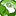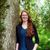# App Development

Discussion board where members can learn more about Qlik Sense App Development and Usage.

Announcements
Welcome to Qlik Community! Check out our new navigation! FIND OUT MORE
cancel
Showing results for
Did you mean:Contributor II

## Accumulative sum by 2 dimensions

I'm trying to get a cumulative month to month sum on a table which is grouped by customer and year.

The idea is to sum "Total" value each month and then subtract that accumulated amount from the "CommittedSpend" column, which is the same for each year.

In the example below, first year of committed spent was \$612,000 and the actual spent was \$123,892 so the calculation was \$612,000-\$123,892=487,107.

On the second month, the spent was \$175,997 and the accumulated spent was \$300,890(124,892+175,995). Then the remaining was the subtraction of \$612,000-\$300,890 giving a result of 311,109, and so on.

This calculation should end on the 12th month of each year and then start again on the next year(if applied) for each customer.

I was using the code below, but that works only when a select a customer from a filter but it stops working if no selection is made.

`` [CommittedSpend]-RANGESUM(ABOVE(TOTAL SUM(Total),0,12))``Labels (3)

• ### Rangesum() Function

1 Solution

Accepted SolutionsMVP

The TOTAL keyword in the above() is telling Qlik to ignore all of your dimensions.  Since you want the dimensions taken into account with the cumulative sum, you cannot use the TOTAL function.  The following expression should work, but you will need to make sure your dimensions are ordered properly and the sorting is in the proper order:

`rangesum(above(sum(Total), 0, RowNo()))`
2 RepliesMVP

The TOTAL keyword in the above() is telling Qlik to ignore all of your dimensions.  Since you want the dimensions taken into account with the cumulative sum, you cannot use the TOTAL function.  The following expression should work, but you will need to make sure your dimensions are ordered properly and the sorting is in the proper order:

`rangesum(above(sum(Total), 0, RowNo()))`Contributor II
Author

This works properly.

Thank you Nicole!Tags
Community Browser